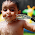### Data Roles: Dimension vs. Measure

Dimensions
Dimensions typically produce headers when added to the rows or columns shelves in the view. By default, Tableau treats any field containing qualitative, categorical information as a dimension. This includes, for instance, any field with text or dates values. However, in relational data sources, the actual definition of a dimension is slightly more complex. A dimension is a field that can be considered an independent variable.
This means that a measure can be aggregated for each value of the dimension. For instance, you might calculate the Sum of “Sales” for every “State”. In this case the State field is acting as a dimension because you want to aggregate sales for each state. The values of Sales are dependent on the State, so State is an independent field and Sales is a dependent field.
Such aggregation could also be computed for numeric fields that are treated as dimensions. For instance, you might want to calculate the SUM of Sales for each “Discount Rate” offered to customers. In this case the Discount Rate field acts as an independent field and the Sales field is dependent even though both fields are numeric. You can use a numeric field as the independent field by first converting the Discount Rate measure to a dimension.
Measures
Measures typically produce axes when added to the rows or columns shelves. By default, Tableau treats any field containing numeric (quantitative) information as a measure. However, in relational data sources, the actual definition of a measure is slightly more complex. A measure is a field that is a dependent variable; that is, its value is a function of one or more dimensions.
This means that a measure is a function of other dimensions placed on the worksheet. For instance, you might calculate the Sum of “Sales” for every “State”. In this case, the Sales field is acting as a measure because you want to aggregate the field for each state. But measures could also result in a non-numeric result. For instance, you might create a calculated measure called “Sales Rating” that results in the word “Good” if sales are good and “Bad” otherwise. In this case the “Sales Rating” field acts as a measure even though it produces a non-numeric result. It is considered a measure because it is a function of the dimensions in the view.

## Converting Measures to Dimensions

By default, Tableau treats all relational fields containing numbers as measures. However, you might decide that some of these fields should be treated as dimensions. For example, a field containing ages may be categorized as a measure by default in Tableau because it contains numeric data. However, if you want to look at each individual age rather than an axis you can convert the Age field to a dimension.
• Click and drag the field from the measures area of the Data window and drop it into the dimensions area.• Right-click the measure in the Data window and select Convert to Dimension.
The Order Quantity field is now displayed in the Dimensions area of the Data window and is a discrete quantity as indicated by the blue number icon.If you place the converted field on a shelve, it produces headers instead of an axis.1.2.3.